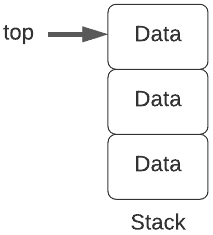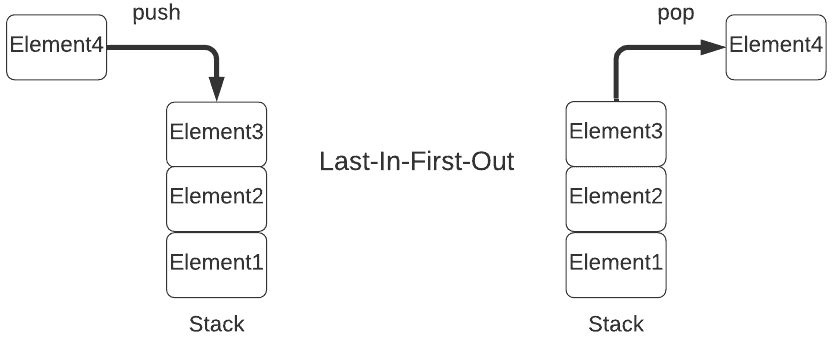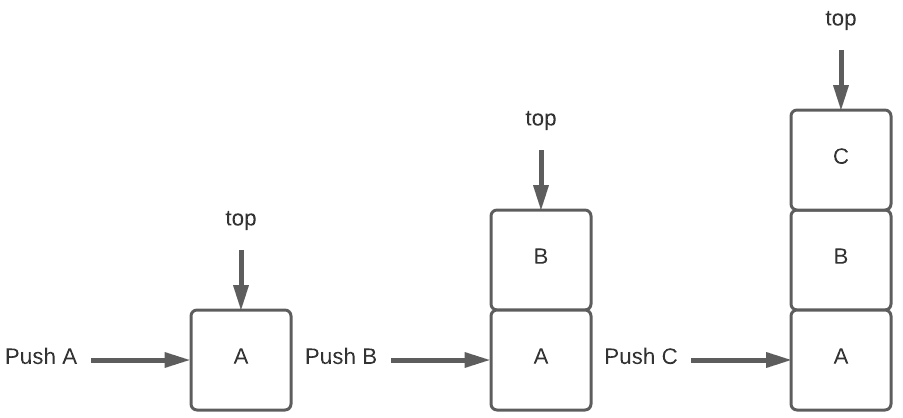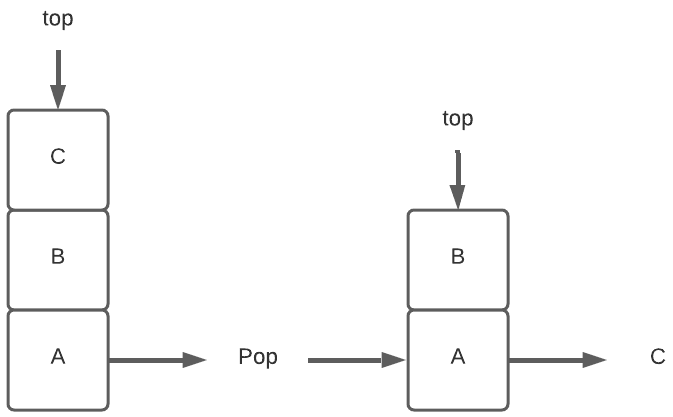## 1. Introduction

In this tutorial, we’ll learn what a stack is and understand how it works. Moreover, we’ll present a general description of the stack data structure and its fundamental operations.

## 2. Stack

Stacks appear in daily life in a lot of places. For example, a stack of books, plates, discs in a disc holder. Let’s take the example of a stack of books. It allows you to perform operations like inserting a book or removing a book only from one end of the stack.

Similarly, a stack is a list in which insertions and deletions are allowed only at one end of the list. This end is called the top of the stack.

Since the element at the top of the stack is the most recently inserted element using the insert operation, and it is also the one to be removed first by the delete operation, the stack is called a Last In First Out (LIFO) list.

In other words, it means that the element which is inserted first into the stack is also the one to be removed last from the stack. Hence, the stack is also called a First In Last Out (FILO) list.Stacks are useful for any application requiring LIFO storage. For instance, parsing context-free languages, evaluating arithmetic expressions, and function call management.

## 3. Representation

Further, let’s look at the representation of the stack.

The below diagram depicts the representation of the stack in memory. As mentioned earlier, stacks follow LIFO order, and you can only access the top of the stack.Next, let’s look at the fundamental operations that we can perform on a stack.

## 4. Operations

The common operations that we can perform on a stack include insertion, deletion, peek and check if the stack is full or empty.

Let’s look at the pseudocode of all these operations.

### 4.1. push()

Firstly, let’s look at the insert operation. Inserting a new element to the top of the stack is known as the push operation.

The following pseudocode shows the details of the push operation:

``````

Let’s look at an example of inserting A, B, and C onto an empty stack. First, we push A and the top points at A. Next, when we push B onto the stack, the top is updated to point at B. Finally, when we push C onto the stack, the top is updated to point at C:### 4.2. pop()

Secondly, let’s look at the delete operation. Deleting an element from the top of the stack is known as the pop operation.

The following pseudocode shows the details of the pop operation:

``

Let’s look at an example of removing the top element from the stack containing A, B, and C:We can see that once the element at the top is removed, i.e., C, the top starts pointing at B.

### 4.3. peek()

Thirdly, the peek operation retrieves the element at the top of the stack without removing it from the stack.

The following pseudocode shows the details of the peek operation:

```freestar.config.enabled_slots.push({ placementName: "baeldung_incontent_1", slotId: "baeldung_incontent_1" });

```

### 4.4. isFull()

It checks whether or not the stack is complete.

The following pseudocode shows the details of the isFull operation:

``

### 4.5. isEmpty()

Finally, let’s look at the isEmpty operation. It checks whether or not the stack is empty.

The following pseudocode shows the details of the isEmpty operation:

``

## 5. Time Complexity Analysis

The stack follows LIFO order for all operations, which means the top is always pointing at the top-most element. Hence, the time complexity for all the common operations is O(1).

## 6. Conclusion

To sum up, we discussed the data structure called a stack. We’ve learned the basic definition of a stack. Furthermore, we’ve learned the basic features and common operations of a stack. Finally, we looked at the pseudocodes to implement these common operations.